# INDEX and MATCH Functions – Multiple Criteria VLOOKUPs

In an ideal world, we would always receive the data we need in exactly the format we need to have it in. However, in the real world, analysts are often given dumps of data from various sources that need to be consolidated into a useful report. Many times, this consolidation is done in Excel using the VLOOKUP function. A limit of the VLOOKUP function, however, is that it can only be used to join data on a single criterion. A less commonly used way to join the data together is through a combination of the INDEX AND MATCH functions. The INDEX/MATCH method can be used when we need to join the data on single or multiple criteria, making it more flexible than the VLOOKUP function.

Following is a case where we need to join data on multiple criteria. Suppose our company is a Natural Gas producer and uses its budgeted Ethane (C2) and Propane (C3) volumes to hedge against price fluctuations. We are asked to build a report showing the Actuals-to-Budget variance of our sales volumes by meter by month for our six (6) highest volume meters. We have built the structure of our report and updated the Budgeted volumes as well as the formulas to calculate the variances. All that we need to complete the report are the Actual volumes.

## Using INDEX and MATCH Functions In an Actuals-to-Budget Report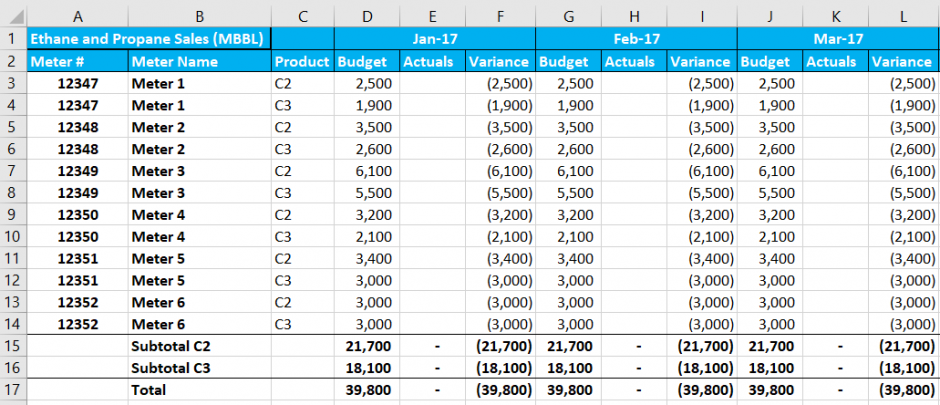Actuals Data:

For the Actuals Data, we receive a dump of all NGL sales for all the company’s meters and all months.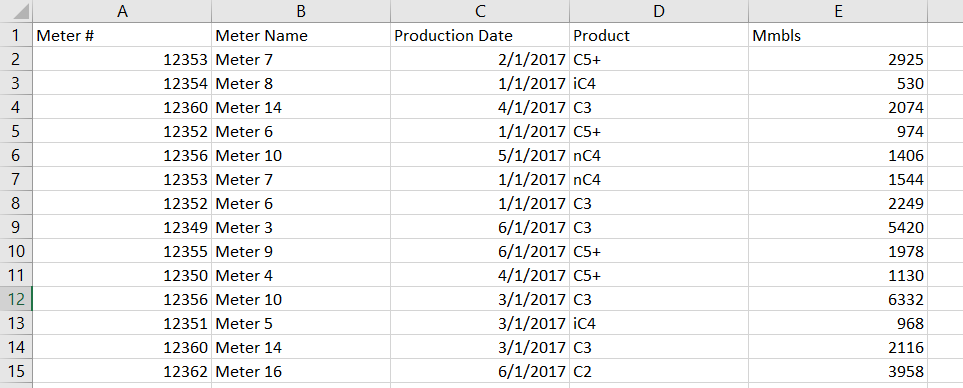To bring the Actuals data accurately into our report, we need to join the data on not only the Meter #, but also on the Product and the Month. A VLOOKUP would be able to join the data on any one of these criteria, but not all three. To join on all three criteria, we can use the Index/Match option.

First, let’s break down the Index function and Match functions separately.

INDEX Function (array, row_num, [column_num]):

With the INDEX Function, we specify a range of data and a row and column number within that range (where the upper left cell of the range is in row 1 and column 1). The INDEX function will return the cell where the specified row and column numbers intersect.  For example, below we have set our range to be A1:E541 which is the entire set of our data dump. We then specify it to return the value in Row 3, Column 5. This value is equal to 530.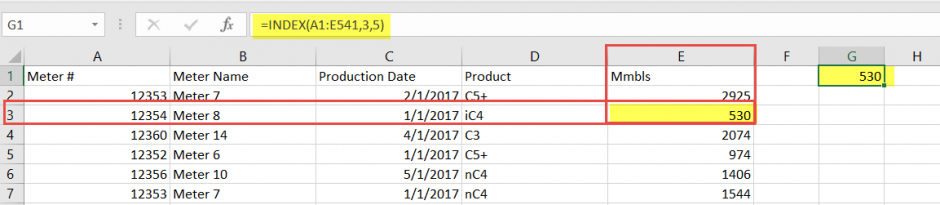The INDEX function gives us a way to return an individual cell from a range. To make this function more valuable though, we need a way to find a specific row of data based on the data required in our report. To accomplish this, we can use the MATCH function.

MATCH Function (lookup_value, lookup_array, [match_type]}:

The MATCH function allows us to enter the value (lookup_value) we want to join and the range (lookup_array) where we want to find that value. For example, below we told the MATCH function to find Meter # 12354 (lookup_value) within range A1:A541 (lookup_array). The function returns number 3 which is the row within the range where Meter # 12354 is first encountered.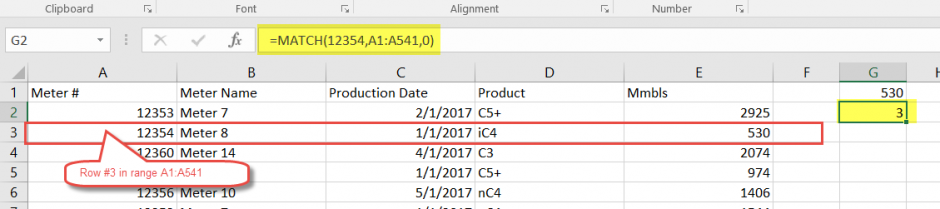Rather than returning the row number with the first occurrence of Meter #12354, suppose we want to return the row for Meter #12354 with product C3 in Production Date 03/01/2017. In other words, we need to return the row number base on multiple criteria.

We can accomplish this by using an Array formula. Specifically, we can use the “&” symbol to join multiple lookup_values and multiple lookup_arrays. One important caveat is that any time you create or edit an Array formula, you must type Ctlr+Shift+Enter in the formula bar to evaluate it. After typing Ctrl+Shift+Enter, curly brackets will appear on the outside of your formula. If you only type Enter after editing an Array formula, a #VALUE! Error will be returned.

Below is a screenshot showing the use of an Array Match formula to identify the desired row based on Multiple Criteria. Note the curly brackets on the outside indicating that it is an Array formula.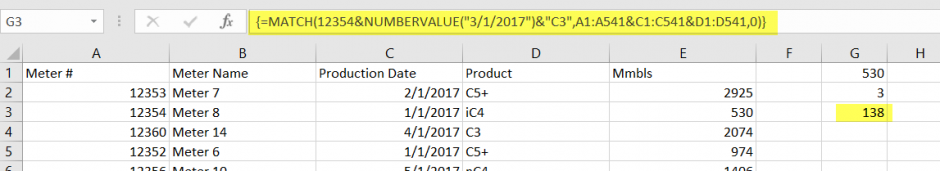The formula returned row number 138. When we look, we can see that row 138 does in fact match all 3 of our criteria: Meter #=12354, Production Date=3/1/2017, and Product=C3.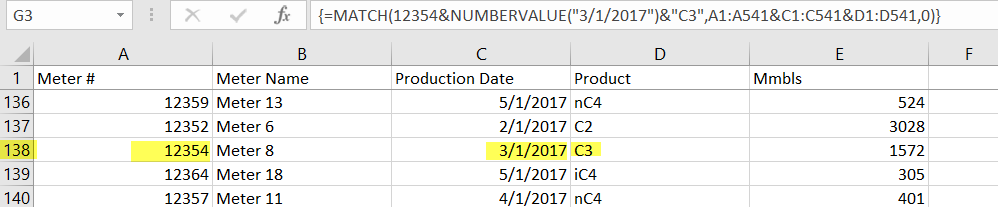Populating Actuals in our Report (INDEX/MATCH combination):

Now that we have a way to return a value from a row/column intersection (INDEX) and a way to identify specific rows of data (MATCH), we can combine the two functions to bring the Actuals data accurately into our report. Since our goal is to return the data within certain cells, we start with the INDEX function and specify the column array where the data we want to return is located. In this case, the data we want to return is Mbbls (thousands of barrels), and these values are in range AA4:AA544.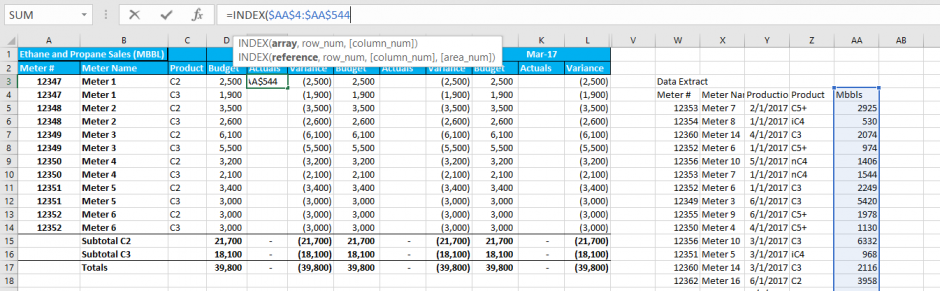Next, we need to specify the row number for the INDEX function. Instead of specifying a specific number, we can nest an Array MATCH function inside the INDEX function to identify the row number based on our 3 criteria: Meter #, Product, and Month.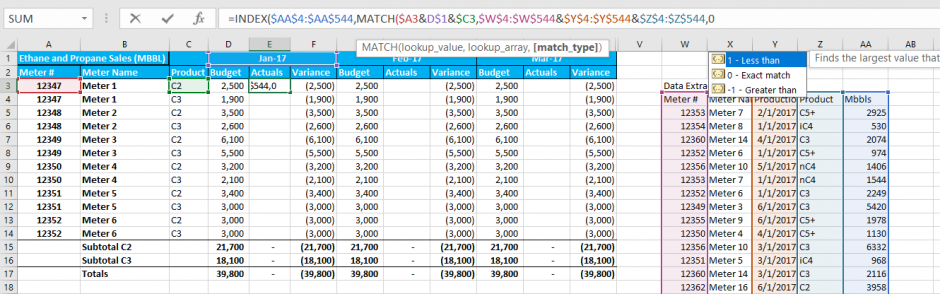We use a combination of Relative and Absolute references (\$ symbol) to make sure our formulas will reference the correct cells once we copy them.

Since we only have one column in the Array for our INDEX function, specifying the column number is optional. Here we will explicitly specify it equal to number 1.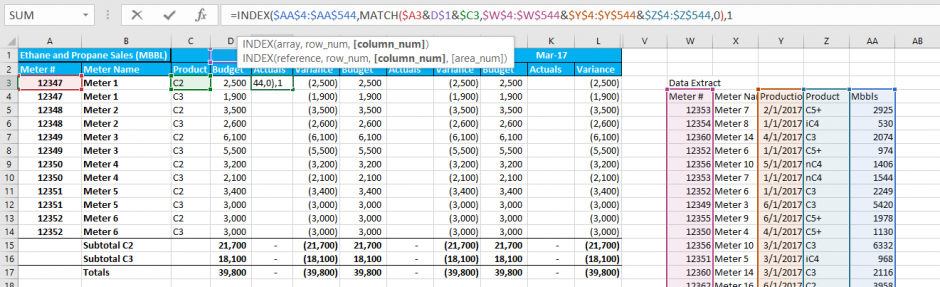We have entered all the arguments for the INDEX function, so we close the final parentheses and press Ctrl+Shift+Enter to evaluate the Array formula. We know we did this correctly because the curly braces appear around our formula.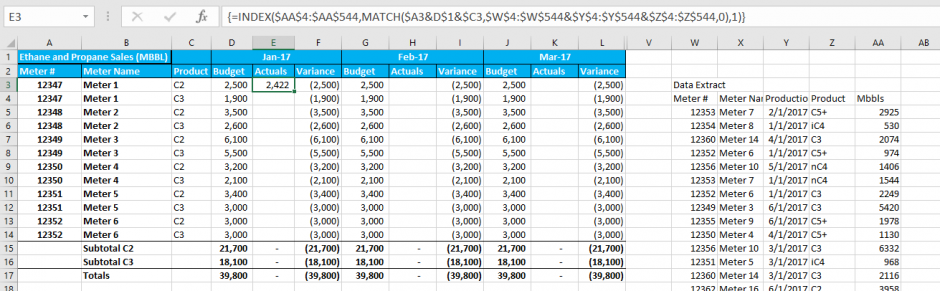The formula returned 2,422 MBBLs of C2 for Meter #12347 in January. Searching through our raw data, we can verify that the formula did return the desired value.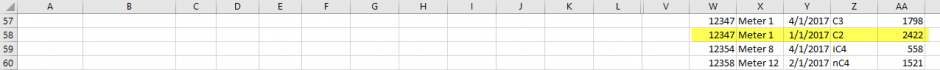We can now simply copy our formulas through the rest of the Actuals columns, and our Actuals-to-Budget Variance report will be complete!

We see that through March, we have exceeded our C2 budgeted volumes on our highest volume meters, but we have fallen short on C3 volumes. The largest drivers of these variances are Meter 3 and Meter 6.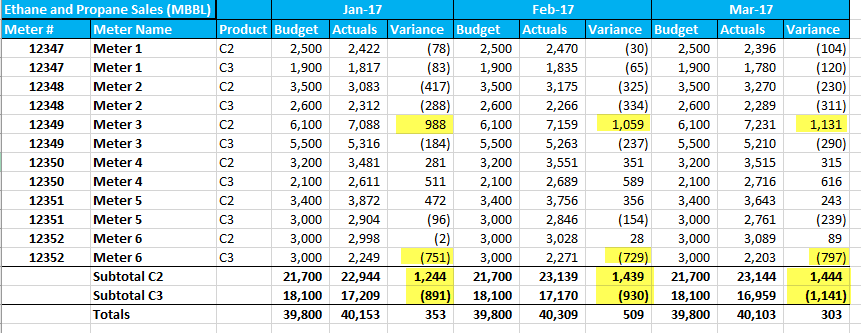## Still Need Help With INDEX and MATCH Functions?

In conclusion, the major advantage of the INDEX/MATCH method is the ability to lookup values on multiple criteria. Using this method can be very helpful for quickly bringing raw data into monthly or ad hoc reports that have been built in Excel.